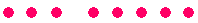S k i l l
i n
A R I T H M E T I C

Prologue 1

# ELEMENTARY ADDITION

Sums less than 10

Composing 10 itself

Sums between 10 and 20

Doubling

Zero

Practice problems

SKILL IN ARITHMETIC begins with knowing sums that are less than 10.  3 + 4 = 7,  7 + 2 = 9, and so on.

First, though, one of the elementary facts of arithmetic is that the order in which we add does not matter.  For if you know that 5 + 3 = 8:then you would also know that 3 + 5 = 8.The order in which we add does not matter.

Now practice these sums less than 10.

To see the answer, pass your mouse over the colored area.
To cover the answer again, click "Refresh" ("Reload").
Do the problem yourself first!

 2 + 7 = 9 3 + 4 = 7 2 + 3 = 5 5 + 2 = 7 1 + 5 = 6 6 + 3 = 9 4 + 2 = 6 3 + 5 = 8 1 + 3 = 4 2 + 4 = 6 1 + 7 = 8 2 + 5 = 7 1 + 8 = 9 4 + 5 = 9 3 + 2 = 5 2 + 6 = 8 1 + 6 = 7 7 + 2 = 9 5 + 3 = 8 4 + 4 = 8 5 + 4 = 9 3 + 6 = 9 1 + 4 = 5 4 + 3 = 7 6 + 2 = 8

Composing 10 itself

It is useful to know all the ways of "composing" -- that is, making -- 10.

 2 + 8 = 10 6 + 4 = 10 1 + 9 = 10 4 + 6 = 10 9 + 1 = 10 7 + 3 = 10 8 + 2 = 10 3 + 7 = 10 5 + 5 = 10

Sums between 10 and 20

Finally, the student must know sums such as  9 + 6 = 15 ,  8 + 5 = 13, and so on.  To become familiar with them, you can first compose 10 by regrouping.  But eventually, you must know them.

Example 1.   9 + 6.

On splitting 6 into 1 + 5,

9 + 6 = 9 + 1 + 5 = 10 + 5 = 15

Say, "9 + 1 is 10, plus 5 is 15."

Regroup 1 with 9 to compose 10.

Example 2.   5 + 7.

"5 + 5 is 10, plus 2 is 12."

Example 3.     3 + 8.

"8 + 2 is 10, plus 1 is 11."

Doubling

It will help to know the sum of a number added to itself.

5 + 5 = 10

6 + 6 = 12

7 + 7 = 14

8 + 8 = 16

9 + 9 = 18

Now, once you know that

6 + 6 = 12,

then you could know that

6 + 7 = 13,

and

6 + 5 = 11.

7 + 7 = 14

7 + 8 = 15

7 + 6 = 13

8 + 8 = 16

8 + 9 = 17

8 + 7 = 15

9 + 9 = 18

9 + 8 = 17

Zero

0 is a number. It can answer the question How much? or How many?
0 is best introduced by subtraction.

Say, for example, that you have 2 dollars.  If you spend those 2 dollars, you now have 0 dollars.

2 dollars − 2 dollars = 0 dollars.

That is,

2 − 2 = 0

for any unit:  dollars, apples, chairs, etc.

Here is a basic fact about 0:  If you add it to any number, that number does not change.

5 + 0 = 5.

0 + 6 = 6.

0 means no units.

There is a subtle difference between 0 units and nothing. Say that you have an account at the First National Bank and that your balance is \$10.  If you now withdraw \$10, your balance is 0 dollars. But say that you have no account at that bank. Then you do not have a balance of 0 dollars there -- you have nothing!

That is the difference between 0 and nothing.

*        *        *

Practice the following until you remember each one.

 8 + 5 = 13 3 + 9 = 12 5 + 9 = 14 6 + 8 = 14 7 + 9 = 16 9 + 6 = 15 7 + 6 = 13 8 + 6 = 14 6 + 5 = 11 8 + 4 = 12 3 + 8 = 11 5 + 8 = 13 9 + 4 = 13 7 + 4 = 11 6 + 6 = 12 4 + 9 = 13 4 + 8 = 12 6 + 9 = 15 8 + 9 = 17 4 + 7 = 11 5 + 7 = 12 6 + 7 = 13 9 + 3 = 12 9 + 2 = 11 7 + 7 = 14 7 + 8 = 15 9 + 5 = 14 9 + 8 = 17 7 + 5 = 12 2 + 9 = 11 9 + 7 = 16 8 + 7 = 15 5 + 6 = 11 8 + 3 = 11 8 + 8 = 16 9 + 0 = 9 8 + 0 = 8 0 + 6 = 6 0 + 3 = 3 0 + 0 = 0

www.proyectosalonhogar.com